Parametric Equalizer Design

This example shows how to design parametric equalizer filters. Parametric equalizers are digital filters used in audio for adjusting the frequency content of a sound signal. Parametric equalizers provide capabilities beyond those of graphic equalizers by allowing the adjustment of gain, center frequency, and bandwidth of each filter. In contrast, graphic equalizers only allow for the adjustment of the gain of each filter.

Typically, parametric equalizers are designed as second-order IIR filters. These filters have the drawback that because of their low order, they can present relatively large ripple or transition regions and may overlap with each other when several of them are connected in cascade. The DSP System Toolbox™ provides the capability to design high-order IIR parametric equalizers. Such high-order designs provide much more control over the shape of each filter. In addition, the designs special-case to traditional second-order parametric equalizers if the order of the filter is set to two.

This example discusses two separate approaches to parametric equalizer design. The first is using designParamEQ and the second is using fdesign.parameq. designParamEQ should serve most needs. It is simpler and provides the ability for most common designs. It also supports C code generation which is needed if there is a desire to tune the filter at run-time with generated code. fdesign.parameq provides many advanced design options for ultimate control of the resulting filter. Not all design options are explored in this example.

Some Basic Designs

Consider the following two designs of parametric equalizers. The design specifications are the same except for the filter order. The first design is a typical second-order parametric equalizer that boosts the signal around 10 kHz by 5 dB. The second design does the same with a sixth-order filter. Notice how the sixth-order filter is closer to an ideal brickwall filter when compared to the second-order design. Obviously the approximation can be improved by increasing the filter order even further. The price to pay for such improved approximation is increased implementation cost as more multipliers are required.

Fs = 48e3;
N1 = 2;
N2 = 6;
G  = 5; % 5 dB
Wo = 10000/(Fs/2);
BW = 4000/(Fs/2);
[B1,A1] = designParamEQ(N1,G,Wo,BW);
[B2,A2] = designParamEQ(N2,G,Wo,BW);
hfvt = fvtool(BQ1,BQ2,'Fs',Fs,'Color','white');
legend(hfvt,'2nd-Order Design','6th-Order Design');One of the design parameters is the filter bandwidth, BW. In the previous example, the bandwidth was specified as 4 kHz. The 4 kHz bandwidth occurs at half the gain (2.5 dB).

Designs Based on Quality Factor

Another common design parameter is the quality factor, Q. The Q of the filter is defined as Wo/BW (center frequency/bandwidth). It provides a measure of the sharpness of the filter, i.e., how sharply the filter transitions between the reference value (0 dB) and the gain G. Consider two designs with same G and Wo, but different Q values.

Fs  = 48e3;
N   = 2;
Q1  = 1.5;
Q2  = 10;
G   = 15; % 15 dB
Wo  = 6000/(Fs/2);
BW1 = Wo/Q1;
BW2 = Wo/Q2;
[B1,A1] = designParamEQ(N,G,Wo,BW1);
[B2,A2] = designParamEQ(N,G,Wo,BW2);
hfvt = fvtool(BQ1,BQ2,'Fs',Fs,'Color','white');
legend(hfvt,'Q = 1.5','Q = 10');Although a higher Q factor corresponds to a sharper filter, it must also be noted that for a given bandwidth, the Q factor increases simply by increasing the center frequency. This might seem unintuitive. For example, the following two filters have the same Q factor, but one clearly occupies a larger bandwidth than the other.

Fs  = 48e3;
N   = 2;
Q   = 10;
G   = 9; % 9 dB
Wo1 = 2000/(Fs/2);
Wo2 = 12000/(Fs/2);
BW1 = Wo1/Q;
BW2 = Wo2/Q;
[B1,A1] = designParamEQ(N,G,Wo1,BW1);
[B2,A2] = designParamEQ(N,G,Wo2,BW2);
hfvt = fvtool(BQ1,BQ2,'Fs',Fs,'Color','white');
legend(hfvt,'BW1 = 200 Hz; Q = 10','BW2 = 1200 Hz; Q = 10');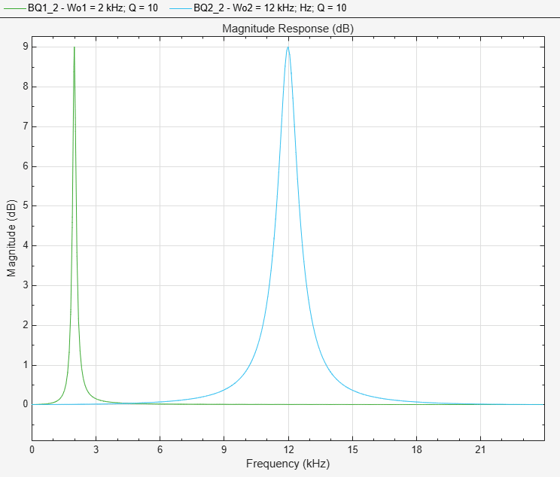When viewed on a log-frequency scale though, the "octave bandwidth" of the two filters is the same.

hfvt = fvtool(BQ1,BQ2,'FrequencyScale','log','Fs',Fs,'Color','white');
legend(hfvt,'Fo1 = 2 kHz','Fo2 = 12 kHz');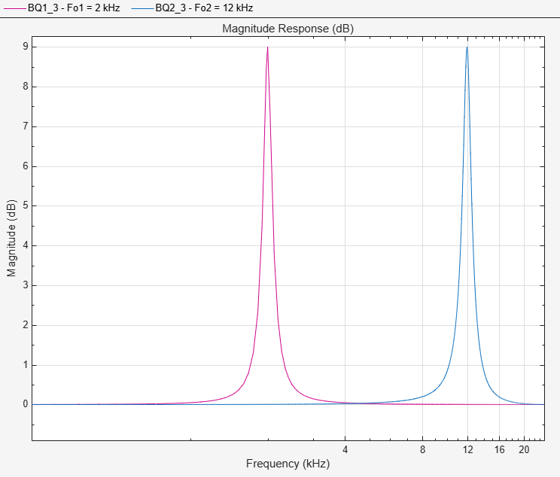Low Shelf and High Shelf Filters

The filter's bandwidth BW is only perfectly centered around the center frequency Wo when such frequency is set to 0.5*pi (half the Nyquist rate). When Wo is closer to 0 or to pi, there is a warping effect that makes a larger portion of the bandwidth to occur at one side of the center frequency. In the edge cases, if the center frequency is set to 0 (pi), the entire bandwidth of the filter occurs to the right (left) of the center frequency. The result is a so-called shelving low (high) filter.

Fs  = 48e3;
N   = 4;
G   = 10; % 10 dB
Wo1 = 0;
Wo2 = 1; % Corresponds to Fs/2 (Hz) or pi (rad/sample)
BW = 1000/(Fs/2); % Bandwidth occurs at 7.4 dB in this case
[B1,A1] = designParamEQ(N,G,Wo1,BW);
[B2,A2] = designParamEQ(N,G,Wo2,BW);
hfvt = fvtool(BQ1,BQ2,'Fs',Fs,'Color','white');
legend(hfvt,'Low Shelf Filter','High Shelf Filter');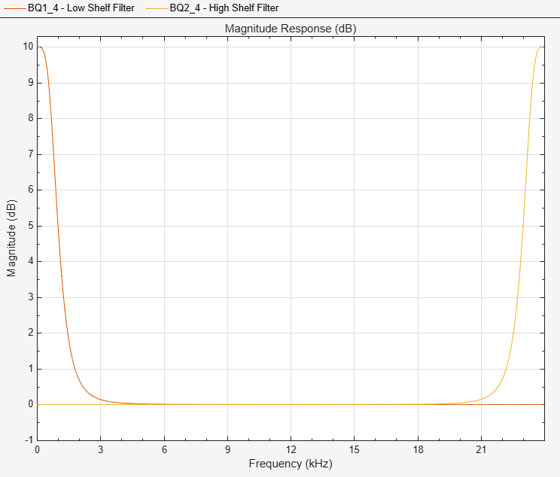A Parametric Equalizer That Cuts

All previous designs are examples of a parametric equalizer that boosts the signal over a certain frequency band. You can also design equalizers that cut (attenuate) the signal in a given region.

Fs = 48e3;
N  = 2;
G  = -5; % -5 dB
Wo = 6000/(Fs/2);
BW = 2000/(Fs/2);
[B,A] = designParamEQ(N,G,Wo,BW);
hfvt = fvtool(BQ,'Fs',Fs,'Color','white');
legend(hfvt,'G = -5 dB');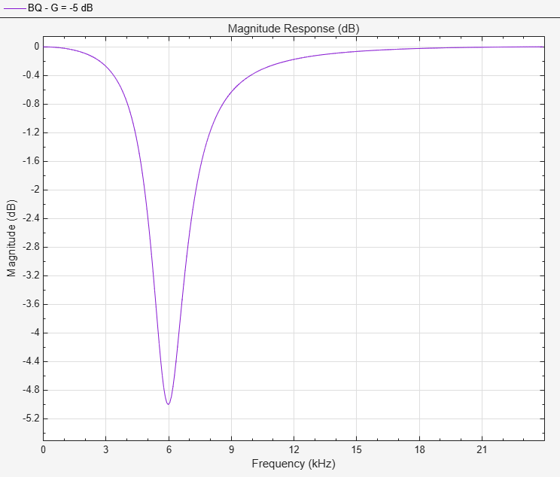At the limit, the filter can be designed to have a gain of zero (-Inf dB) at the frequency specified. This allows to design 2nd order or higher order notch filters.

Fs  = 44.1e3;
N   = 8;
G   = -inf;
Q   = 1.8;
Wo  = 60/(Fs/2); % Notch at 60 Hz
BW  = Wo/Q; % Bandwidth will occur at -3 dB for this special case
[B1,A1] = designParamEQ(N,G,Wo,BW);
[NUM,DEN]  = iirnotch(Wo,BW); % or [NUM,DEN] = designParamEQ(2,G,Wo,BW);
SOS2 = [NUM,DEN];
hfvt = fvtool(BQ1,BQ2,'Fs',Fs,'FrequencyScale','Log','Color','white');
legend(hfvt,'8th order notch filter','2nd order notch filter');Parametric equalizers are usually connected in cascade (in series) so that several are used simultaneously to equalize an audio signal. To connect several equalizers in this way, use the dsp.FilterCascade.

Fs  = 48e3;
N   = 2;
G1  = 3;  % 3 dB
G2  = -2; % -2 dB
Wo1 = 400/(Fs/2);
Wo2 = 1000/(Fs/2);
BW = 500/(Fs/2); % Bandwidth occurs at 7.4 dB in this case
[B1,A1] = designParamEQ(N,G1,Wo1,BW);
[B2,A2] = designParamEQ(N,G2,Wo2,BW);
hfvt = fvtool(FC,'Fs',Fs,'Color','white','FrequencyScale','Log');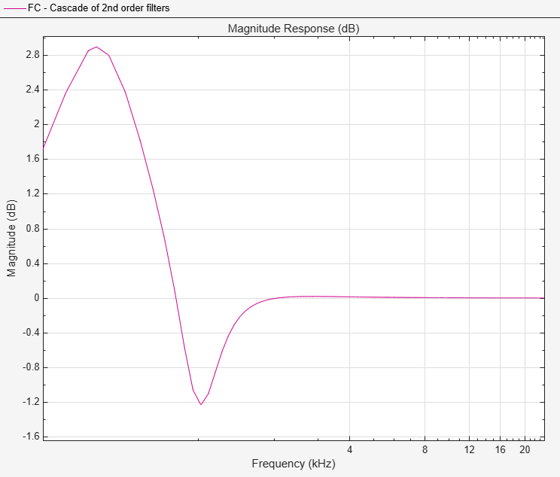Low-order designs such as the second-order filters above can interfere with each other if their center frequencies are closely spaced. In the example above, the filter centered at 1 kHz was supposed to have a gain of -2 dB. Due to the interference from the other filter, the actual gain is more like -1 dB. Higher-order designs are less prone to such interference.

Fs  = 48e3;
N   = 8;
G1  = 3;  % 3 dB
G2  = -2; % -2 dB
Wo1 = 400/(Fs/2);
Wo2 = 1000/(Fs/2);
BW = 500/(Fs/2); % Bandwidth occurs at 7.4 dB in this case
[B1,A1] = designParamEQ(N,G1,Wo1,BW);
[B2,A2] = designParamEQ(N,G2,Wo2,BW);
hfvt = fvtool(FC,FC2,'Fs',Fs,'Color','white','FrequencyScale','Log');Advanced Design Options: Specifying Low and High Frequencies

For more advanced designs, fdesign.parameq can be used. For example, because of frequency warping, in general it can be difficult to control the exact frequency edges at which the bandwidth occurs. To do so, the following can be used:

Fs    = 44.1e3;
N     = 4;
Flow  = 3000;
Fhigh = 4000;
Grsq  = 1;
Gref  = 10*log10(Grsq);
G     = -8;
Gsq   = 10.^(G/10); % Magnitude squared of filter; G = 5 dB
GBW   = 10*log10((Gsq + Grsq)/2);  % Flow and Fhigh occur at -2.37 dB

PEQ = fdesign.parameq('N,Flow,Fhigh,Gref,G0,GBW',...
N,Flow/(Fs/2),Fhigh/(Fs/2),Gref,G,GBW);
BQ = design(PEQ,'SystemObject',true);
hfvt = fvtool(BQ,'Fs',Fs,'Color','white');
legend(hfvt,'Equalizer with Flow = 3 kHz and Fhigh = 4 kHz');Notice that the filter has a gain of -2.37 dB at 3 kHz and 4 kHz as specified.

Shelving Filters with a Variable Transition Bandwidth or Slope

One of the characteristics of a shelving filter is the transition bandwidth (sometimes also called transition slope) which may be specified by a shelf slope parameter S. The bandwidth reference gain GBW is always set to half the boost or cut gain of the shelving filter. All other parameters being constant, as S increases the transition bandwidth decreases, (and the slope of the response increases) creating a "slope rotation" around the GBW point as illustrated in the example below.

N  = 2;
Fs = 48e3;
Fo = 0;  % F0=0 designs a lowpass filter, F0=1 designs a highpass filter
Fc = 2e3/(Fs/2); % Cutoff Frequency
G  = 10;

S  = 1.5;
PEQ = fdesign.parameq('N,F0,Fc,S,G0',N,Fo,Fc,S,G);
BQ1 = design(PEQ,'SystemObject',true);

PEQ.S = 2.5;
BQ2 = design(PEQ,'SystemObject',true);

PEQ.S = 4;
BQ3 = design(PEQ,'SystemObject',true);

hfvt = fvtool(BQ1,BQ2,BQ3,'Fs',Fs,'Color','white');
legend(hfvt,'S=1.5','S=2.5','S=4');The transition bandwidth and the bandwidth gain corresponding to each value of S can be obtained using the measure function. We verify that the bandwidth reference gain GBW is the same for the three designs and we quantify by how much the transition width decreases when S increases.

m1 = measure(BQ1);
get(m1,'GBW')
ans = 5
m2 = measure(BQ2);
get(m2,'GBW')
ans = 5
m3 = measure(BQ3);
get(m3,'GBW')
ans = 5
get(m1,'HighTransitionWidth')
ans = 0.0945
get(m2,'HighTransitionWidth')
ans = 0.0425
get(m3,'HighTransitionWidth')
ans = 0.0238

As the shelf slope parameter S increases, the ripple of the filters also increases. We can increase the filter order to reduce the ripple while maintaining the desired transition bandwidth.

N   = 2;
PEQ = fdesign.parameq('N,F0,Fc,S,G0',N,Fo,Fc,S,G);
BQ1 = design(PEQ,'SystemObject',true);

PEQ.FilterOrder = 3;
BQ2 = design(PEQ,'SystemObject',true);

PEQ.FilterOrder = 4;
BQ3 = design(PEQ,'SystemObject',true);

hfvt = fvtool(BQ1,BQ2,BQ3,'Fs',Fs,'Color','white');
legend(hfvt,'N=2','N=3','N=4');Shelving Filters with a Prescribed Quality Factor

The quality factor Qa may be used instead of the shelf slope parameter S to design shelving filters with variable transition bandwidths.

N  = 2;
Fs = 48e3;
Fo = 1;  % F0=0 designs a lowpass filter, F0=1 designs a highpass filter
Fc = 20e3/(Fs/2); % Cutoff Frequency
G  = 10;
Q  = 0.48;
PEQ = fdesign.parameq('N,F0,Fc,Qa,G0',N,Fo,Fc,Q,G);
BQ1 = design(PEQ,'SystemObject',true);

PEQ.Qa = 1/sqrt(2);
BQ2 = design(PEQ,'SystemObject',true);

PEQ.Qa = 2.0222;
BQ3 = design(PEQ,'SystemObject',true);

hfvt = fvtool(BQ1,BQ2,BQ3,'Fs',Fs,'Color','white');
legend(hfvt,'Qa=0.48','Qa=0.7071','Qa=2.0222');Audio Toolbox DocumentationGet trial now